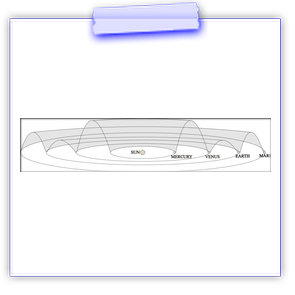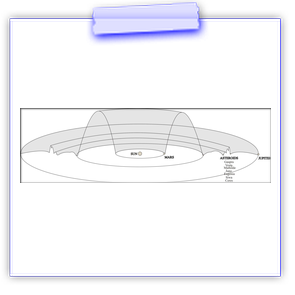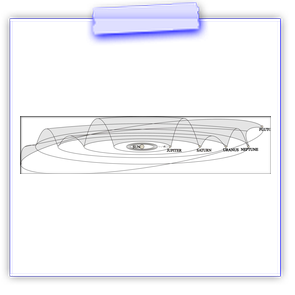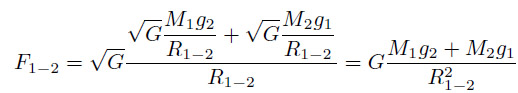# tsiganok.cc.uaTSIGANOK GRAVITATIONAL THEORY (TGT)Obtained using the new formula for the gravitational force between bodies is significantly different from the force in Newton's formula:

• to new formula gives the dimension of force without taking into account gravitational constant;

• masses of the bodies being attracted are not multiplied by each other but they are multiplied by the respective gravity accelerations and are summed up after that;

• constant includes only those quantity dimensions which are contained in the new gravitation formula.

The main results obtained using a Tsiganok gravitation theory (TGT) (the new gravitation theory (the NGT)

Recent publications:

1. Tsiganok E.P., Tsiganok O.E. The determination of fundamental physical constants (FPC) in Tsiganok gravitational theory (TGT) (The article will soon be ready for publication. The estimated volume is about 600 pages.)

2. Tsiganok E.P., Tsiganok O.E. About Tsiganok gravitation theory (TGT) J Astrophys Aerospace Technol 3: 109. doi:10.4172/2329-6542.1000109 (http://bit.ly/1CAmhev) (http://bit.ly/1PvN8bz) (http://tsiganok.cc.ua/)

3.
Tsiganok E.P., Tsiganok O.E. Measuring gravitational constant and the main the Earth, the Sun and the Moon parameters // Nauka i Studia, No. 2, 2009

4. Tsiganok E.P., Tsiganok O.E. Measuring the main physical and astrophysical constants and other bodies parameters //Nauka i Studia, No. 5, 2008

5.
Tsiganok E.P., Tsiganok O.E. Measuring gravitational waves parameters and the mechanism of theformation of different bodies // Nauka i Studia, No. 11, 2009

And also:

Monograph "The laws of motion of bodies" (draft)

Graphs of gravitational waves generated by different bodies in the solar system